Applied and Computational Mathematics
Volume 4, Issue 4, August 2015, Pages: 331-334

The General Forms of the Multiple-Soliton Solutions for the Completely Integrable Equations by Using the Simplest Equation Method

Sen-Yung Lee1, Chun-Ku Kuo1, 2, *

1Department of Mechanical Engineering, National Cheng Kung University, Taiwan, R.O.C.

2Department of Mechanical Engineering, Air Force Institute of Technology, Taiwan, R.O.C.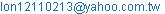(Chun-Ku K.)

Sen-Yung Lee, Chun-Ku Kuo. The General Forms of the Multiple-Soliton Solutions for the Completely Integrable Equations by Using the Simplest Equation Method. Applied and Computational Mathematics. Vol. 4, No. 5, 2015, pp. 331-334. doi: 10.11648/j.acm.20150404.21

Abstract: The simplest equation method with the Burgers’ equation as the simplest equation is used to handle two completely integrable equations, the KdV equation and the potential KdV equation. The general forms of the multiple-soliton solutions are formally established. It is shown that the simplest equation method may provide us with a straightforward and effective mathematic tool for generating multiple-soliton solutions of nonlinear wave equations in fluid mechanics.

Keywords: The Simplest Equation Method, Burgers’ Equation, KdV, The Potential KdV, Multiple-Soliton Solutions

1. Instruction

It is well known that the nonlinear physical phenomena are related to nonlinear partial differential equations which are involved in many fields such as physics, chemistry, mechanics, etc. As mathematical models of the phenomena, the investigation of exact solutions of the partial differential equations will help one to understand these phenomena better. In recent years, many powerful methods to construct exact solutions of nonlinear partial differential equations have been established and developed. Among these are the modified extended tanh-function method , the Exp-function method [2-3], the tanh-coth method , sine-cosine method , Hirota’s direct method [6-7], the F-expansion method , the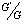-expansion method , and the simplest equation method [10-16].

The above methods can be used to handle the nonlinear wave equations for single soliton solutions, and Guo et al.  think the multiple-soliton solutions of the nonlinear wave equations can be obtained only by three well-known methods : the inverse scattering method, the Backlund transformation method, and the Hirota method. However, we find that the simplest equation method with the Burgers’ equation as the simplest equation is ideal for the determination of multiple-soliton solutions for a wide class of the nonlinear wave equation.

In this paper, we present the simplest equation method to construct the multiple-soliton solutions for the KdV equation and the potential KdV equation .

The rest of this paper is organized as follows. In Section 2, the simplest equation method for finding multiple-soliton solutions of the nonlinear wave equation is described. In Section 3, the method to solve the KdV equation and the potential KdV equation is illustrated in detail. The general forms of the multiple-soliton solutions are obtained. In Section 4, some conclusions are given.

2. The Simplest Equation Method

Let us have a partial differential equation and let by means of an appropriate ansatz this equation be reduced to a nonlinear ordinary differential equation (ODE)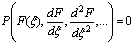(2.1)

For large class of equations from the kind (2.1) exact solution can be constructed as finite series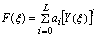(2.2)

where ai are constants to be determined; Y is the exact solution of some ordinary differential equation referred to as the simplest equation; and,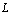is a constant to be determined and the power of the specified solution function finite series, Y. The simplest equation is of lesser order than equation (2.1) and we know the general solution of the simplest equation or we know at least exact analytical particular solutions of the simplest equation .

The application of the simplest equation method is based on the following steps.

1.   By means of the traveling wave ansatz the solved class of nonlinear partial differential equation (NPDE) is reduced to a class of nonlinear ODEs of the kind equation (2.1).

2.   The finite-series solution (2.2) is substituted in (2.1) and as a result a polynomial of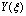is obtained. Equation (2.2) is a solution of (2.1) if all coefficients of the obtained polynomial ofare equal to zero.

3.   By means of a balance equation one ensures that there are at least two terms in the coefficient of the highest power of. The balance equation gives a relationship between the parameters of the solved class of equations and the parameters of the solution.

4.   The application of the balance equation and equalizing the coefficients of the polynomial ofto zero leads to a system of nonlinear relationships among the parameters of the solution and the parameters of the solved class of equation.

5.   Each solution of the obtained system of nonlinear algebraic equations leads to a solution of a nonlinear PDE from the investigated class of nonlinear PDEs.

In order to construct the multiple-soliton solutions for the investigated class of nonlinear PDEs, the Burgers’ equation is chosen as the simplest equation due to that it is a completely integrable equation [4,18].

Consider the Burgers’ equation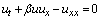(2.3)

where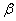is an arbitrary nonlinear coefficient. The multiple-soliton solutions of equation (2.3) is(2.4)

The traveling wave ansatz is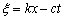, so that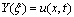. The Burgers’ equation (2.3) is thus transformed into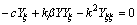(2.5)

Integrating equation (2.5) once with respect to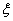, and making the integral constant to zero, it becomes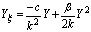(2.6)

It is well known that dispersion relation of the equation (2.3) is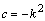(2.7)

Therefore, equation (2.6) is rewritten as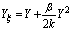(2.8)

and its general form of N-soliton solution is(2.9)

Equation (2.8) is the final form of the simplest equation which will be used to construct the multiple-soliton solutions for the investigated class of nonlinear PDEs.

3. Application

3.1. The General Form of the Multiple-Soliton Solutions for the KdV Equation

Consider the KdV equation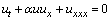(3.1)

where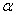is an arbitrary nonlinear coefficient.

Use the traveling wave ansatz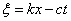to transform equation (3.1) into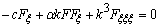(3.2)

Integrating equation (3.2) once with respect to, and making the integral constant to zero, it becomes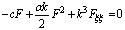(3.3)

Substituting equations (2.2) and (2.8) into (3.3) and by means of the balance equation gives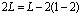(3.4)

Equation (3.4) yields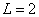. Therefore, the exact solution of equation (3.3) is assumed as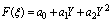(3.5)

Substituting equations (2.8) and (3.5) into (3.3), and setting like power coefficient of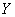to zero, leads a system of nonlinear relationship among the parameters of the solution and the parameters of the solved equation class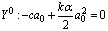(3.6)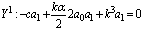(3.7)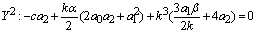(3.8)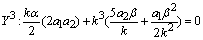(3.9)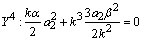(3.10)

Solving equations (3.6-10) yields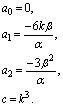(3.11)

As a result the general form of N-soliton solutions is derived as(3.12)

For the one-soliton solution and setting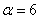, equation (3.12) is the same as the result in Wazwaz’s book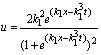(3.13)

3.2. The General Form of the Multiple-Soliton Solutions for the Potential KdV Equation

Consider the potential KdV equation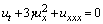(3.14)

where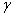is an arbitrary nonlinear coefficient.

Use the traveling wave ansatzto transform equation (3.14) into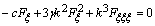(3.15)

Substituting equations (2.2) and (2.8) into (3.15) and processing as before we have. Therefore, the exact solution of equation (3.15) is assumed as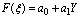(3.16)

Substituting equations (2.8) and (3.16) into (3.15), and setting like power coefficient of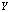to zero, leads a system of nonlinear relationship among the parameters of the solution and the parameters of the solved equation class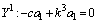(3.17)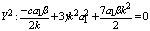(3.18)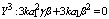(3.19)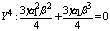(3.20)

Solving equations (3.17-20) yields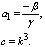(3.21)

Here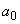is an arbitrary constant, setting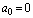.

As a result the general form of N-soliton solutions is derived as(3.22)

For the one-soliton solution and setting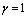, equation (3.22) is the same as the result in Wazwaz’s book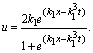(3.23)

Up to now, two completely intrgrable equations are solved by the simplest equation method with the Burgers’ equation as the simplest equation.

4. Conclusions

In this paper, we have presented a concise method to handle two completely integrable equations, the KdV equation and the potential KdV equation. The general forms of the multiple-soliton solutions are formally derived. Not like the inverse scattering method, the Backlund transformation method, and the Hirota method, the results confirm the simplest equation method is concise and direct for constructing multiple-soliton solutions. Therefore, we believe that multiple-soliton solutions existing for other classes of nonlinear mathematic physics models are easily solved. Further work on these aspects is worthy of performing.

Reference

1. Soliman, AA. The modified extended tanh-function method for solving Burgers-type equations. Physica A 361, 394-404 (2006)
2. Ebaid, A. Exact solitary wave for some nonlinear evolution equations via Exp-function method. Phys. Lett. A 365, 213-219 (2007)
3. He, JH.Exp-function method for nonlinear wave equations. Chaos Solitons Fractals 30, 700-708 (2006)
4. Wazwaz, AM. Multipleple-front solutions for the Burgers equation and the coupled Burgers equations. Applied Mathematics and Computation 190, 1198-1206 (2007)
5. Wazwaz, AM. The tanh method and the sine-cosine methods for a reliable treatment of the modified equal width equation and its variants. Communications in Nonlinear Science and Numerical Simulation 11, 148-160 (2006)
6. Hirota, R. The direct method in soliton theory. Cambridge, Cambridge University Press 2004.
7. Hereman, W. Nuseir, A. Symbolic methods to construct exact solutions of nonlinear partial differential equations. Math. Comput. Simulation. 43, 13-27 (1997)
8. Abdou, MA.The extended F-expansion method and its application for a class of nonlinear evolution equations.Chaos Solitons Fractals 31, 95-104 (2007)
9. Zayed, EME.Gepreel, KA.The (G_/G)-expansion method for finding traveling wave solutions of nonlinear partial differential equations in mathematical physics. J. Math. Phys. 50, 013502 (2009)
10. Kudryashov, NA. One method for finding exact solutions of nonlinear differential equations. Commun Nonlinear Sci Numer Simulat 17, 2248-2253 (2012)
11. Vitanov, NK. On modified method of simplest equation for obtaining exact and approximate solutions of nonlinear PDEs:The role of the simplest equation. Commun Nonlinear Sci Numer Simulat 16, 4215-4231 (2011)
12. Kudryashov, NA. Seven common errors in finding exact solutions of nonlinear differential equations. Commun. Nonlinear Sci. Numer. Simulat. 14, 3507-3529 (2009)
13. Vitanov, NK. Application of simplest equations of Bernoulli and Riccati kind for obtaining exact travelling-wave solutions for a class of PDEs with polynominal nonlinearity. Commun Nonlinear Sci Numer Simulat 15, 2050-2060 (2010)
14. Kudryashov, NA. Modified method of simplest equation:Powerful tool for obtaining exact and approximate travelling-wave solutions of nonlinear PDEs. Commun Nonlinear Sci Numer Simulat 16, 1176-1185 (2011)
15. Kudryashov, NA. Loguinova, NB. Extended simplest equation method for nonlinear differential equations. Applied Mathematics and Computation 205, 396-402 (2008)
16. Mohamad, JA. Petkovic, MD. Biswas, A. Modified simple equation method for nonlinear evolution equations. Applied Mathematics and Computation 217, 869-877 (2010)
17. Peng, G. Wu, X. Wang, LB. Multipleple soliton solutions for the variant Boussinesq equations. Advances in Difference Equations 1, 1-11 (2015)
18. Wazwaz, AM. Partial differential equations and solitary waves theory. Springer Science & Business Media, 2010.

 Contents 1. 2. 3. 3.1. 3.2. 4.
Article ToolsAbstractPDF(167K)